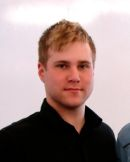# Complexity Classes in Wolfram|Alpha

February 21, 2011 —Comments Off

An algorithm is, in essence, a procedure given by a finite description that solves some computational problem. The field of computational complexity deals with questions of the efficiency of algorithms, i.e. “For a computational problem X, how many steps does the best algorithm perform in solving X?” You might think that questions in this field would be confined to the realm of computer science, except for the fact that computational complexity theory contains the mathematical problem of the century! Currently, many mathematicians around the world are attempting to solve the famed open problem P vs. NP, a problem so important that it is one of the seven millennium problems of the Clay Mathematics Institute and carries a million dollar prize. In fact, according to our logs, many of you tried to ask Wolfram|Alpha this same question before this new functionality was available! But before we talk about how Wolfram|Alpha can help you become a millionaire, let us begin with a historical overview of the subject.

It is generally accepted that the foundations of modern computer science were laid in the 1930s, for it was in this decade that Turing machines, recursive functions, λ-calculus (the inspiration for LISP), Markov algorithms, and many other forms of computation were invented. As it turned out, all of these models had identical computational power (e.g. any Turing machine can be simulated by a random-access machine and vice-versa with polynomial overhead). This observation eventually lead to the “Complexity-Theoretic Church-Turing Thesis”, which states that every physically realizable computational method can be efficiently simulated on a probabilistic Turing machine (with the possible exception of the quantum computer model). After World War II, the von Neumann architecture of EDVAC (one of the first digital computers) gave rise to the revolution of electronic universal computers. As computer use became increasingly widespread, issues of efficiency in computation became of greater concern, and a theory of computational complexity was needed.

Computational complexity is a measure of the resources (e.g. time, space, etc.) that are required to compute a query as a function of input size. Everyone has an intuitive understanding of computational efficiency and the obvious fact that some problems are more difficult than others (perhaps you recall from grade school the difference in difficulty of addition and long division). An important concept in this theory is the notion of a computational complexity class. A complexity class is specified by four parameters: the underlying model of computation, the mode of computation, the resource we wish to bound, and the bounding function itself. We typically assume the model to be a Turing machine. We could indeed choose to measure the complexity of a computation on some other model—say, a lambda expression—but we would obtain exactly the same complexity class, since efficiency is model independent. The two modes of computation usually considered are the deterministic and nondeterministic variations of Turing machines. Lastly, the two resources of greatest interest are time and space, and the complexity function is in principle any function mapping ℕ (the nonnegative integers) to itself.

So all together, a complexity class is a set of computational problems that are solvable by a specific Turing machine M, such that for any input x, M expends at most f(|x|) units of the specified resource. The most famous complexity class is P (model: Turing machine, mode: deterministic, resource: time, bound: polynomial). This popular complexity class was defined by Cobham and attempts to capture mathematically the set of all computational problems that have efficient solution algorithms (the assertion that tractable problems are polynomial-time is called the “Cook-Karp Thesis”). Let us take a look at what Wolfram|Alpha knows about this class by trying “computational complexity class P”: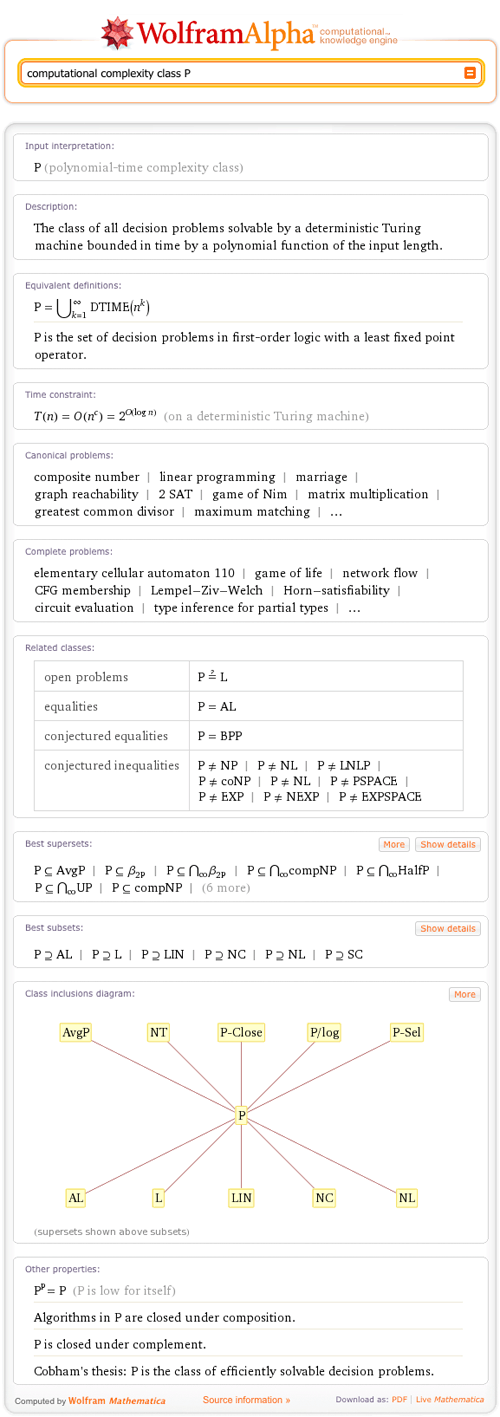Note that if you see a short name for a class that you do not recognize, you can either click it to see a query on that class or click the “Show details” button, which displays the class’s full name. You might also notice that Wolfram|Alpha has given us an “Equivalent definitions” pod, which references a class called DTIME(f(n)). DTIME is the class of problems decided by deterministic Turing machines of time complexity bounded by f(n). This class was defined by Hartmanis and Stearns, who also coined the term “computational complexity” and proved the famous “speed-up theorem”. Now, while in principle, P contains problems that have asymptotic complexity O(n1000) (the running time grows within a constant factor of n1000 where n is the input size), this is not empirically the type of algorithm we tend to find. In fact, problems in P have algorithms that usually run no worse than O(n3) on a random access machine, which would be O(n18) running on a one tape deterministic Turing machine. For example, matrix multiplication can be preformed by Strassen’s algorithm in O(n2.81), and Wolfram|Alpha will give many more noteworthy problems that are contained in this class in addition to complete problems (in the “Complete problems” pod, click the elementary cellular automaton 110 link).

Now, if we group all computational problems by the time required to solve them using the best-known solution algorithm, we get a hierarchy of computational complexity classes. Wolfram|Alpha now provides a way to query this hierarchical zoo in addition to viewing it by showing a helpful graph of relativizing inclusions. For instance, it is a relatively deep theorem with lots of details that the class BPP (bounded-error probabilistic polynomial-time) is contained in P/poly (nonuniform polynomial-time). However, Wolfram|Alpha has access to all this information, and so you can instantly check this result with a single query such as “is BPP contained in P/poly?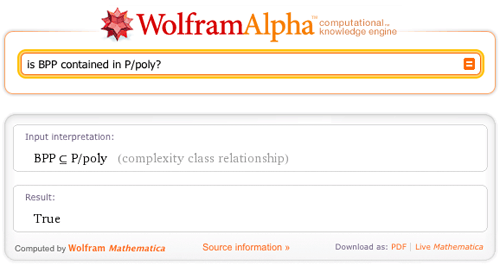No discussion of complexity theory would be complete—no pun intended—without touching on the P vs. NP problem. NP stands for “nondeterministic polynomial time” and refers to the set of problems whose solutions are not necessarily easy to find, but easy to verify.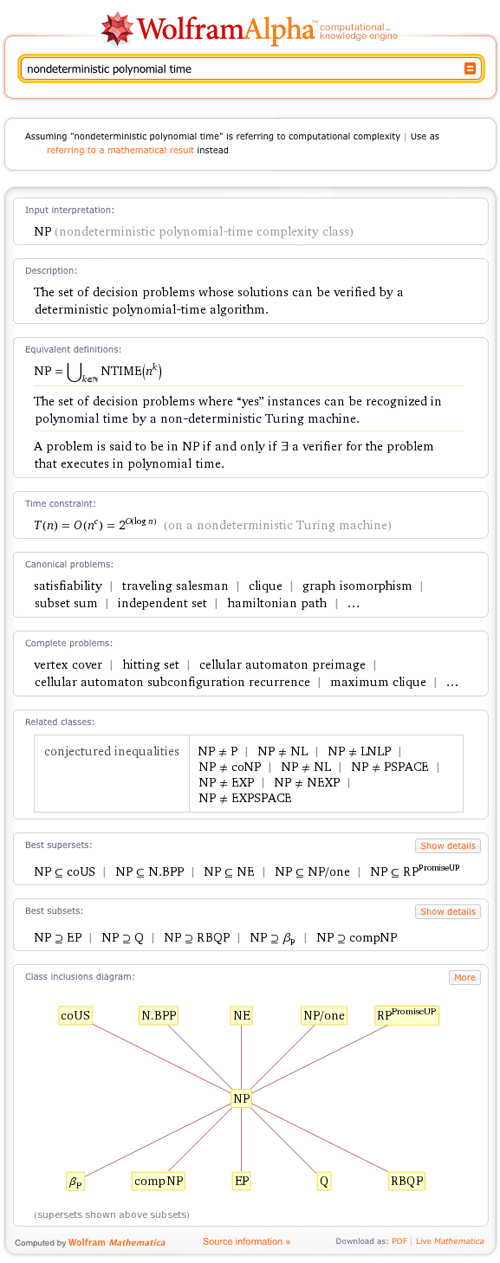In a nutshell, the P vs. NP problem asks whether problems that appear hard to solve actually have easy solutions. This problem is truly a gem, not only of complexity theory, but also of mainstream science. For instance, a literature search on the related concept of NP-completeness (a property of the majority of NP problems), restricted to just physics and chemistry, returns over two thousand papers! Now, if you pay attention to geek news sites like Slashdot, you might be aware of the recent wave of excitement over Hewlett-Packard employee Vinay Deolalikar’s claimed proof of the famous P ≠ NP problem, which means that problems exist that are easier to verify than to solve. However, this paper was found insufficient, and so the search for an answer continues. In the meantime, NP-class problems show up everywhere from Sudoku to trip-scheduling to modern cryptography, factoring huge composite numbers, and thus we must be able to cope with the assumption that P does not equal NP. In response to NP problems, clever workarounds have been designed (approximation algorithms) such as stochastic optimization, which gives imperfect but often sufficient solutions to hard problems.

Even if you cannot prove P ≠ NP, there are many other important research problems in complexity theory to keep you busy! In terms of other related complexity classes, the state of our knowledge is summarized by the chain of inequalities: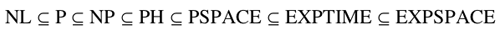We know that NL is a strict subset of PSPACE and that PSPACE is a strict subset of EXPSPACE, which leaves a number of possibilities, all of which are open problems in complexity theory.

To summarize, computational complexity is a fascinating theory with deep connections to mathematics and great implications. Now that its functionality has been added to Wolfram|Alpha, students and experts alike can instantly learn about the properties and interrelationships of both popular and esoteric computational complexity classes!

It doesn’t seem like it knows much or any descriptive complexity. For example, “Is NP contained in SO?” turns up nothing. This is a little surprising to me since Neil Immerman’s book Descriptive Complexity is listed as a reference under “Source information.”

Posted by Jerry February 21, 2011 at 5:13 pm

I don’t believe factoring huge numbers is NP-complete. There’s even a heuristic proof that factoring integers is in P.

Posted by Kedorlaomer February 22, 2011 at 12:04 pm

Great Post Michael. Very Interesting stuff

Posted by Matt February 22, 2011 at 4:23 pm

Unlike almost all other branches of mathematics, a very great number of the most natural problems in complexity theory are not only open, they are undecidable.

A canonical example Emanuele is Viola’s Theorem: Runtimes in P are undecidable. This theorem was recently proved by Viola as the answer to the TCS StackExchange question “Are runtime bounds in P decidable?”

It appears that Wolfram|Alpha has never before grappled with a branch of mathematics in which undecidability is an attribute of problems that arise naturally, as contrasted with artificially.

What are the plans in this regard? Can Wolfram|Alpha recognize other undecidable problems, in complexity theory and elsewhere?

A systematic survey of undecidable problems in complexity theory would be very valuable, as these problems arise in complexity theory more often and more naturally, than in any other branch of mathematics.

Posted by John Sidles February 23, 2011 at 5:52 am

\$P \neq EXP\$ and \$P \neq EXPSPACE\$ are not conjectures. They are known to be true from the time and space hierarchy theorems.

Posted by Manan February 24, 2011 at 11:53 am